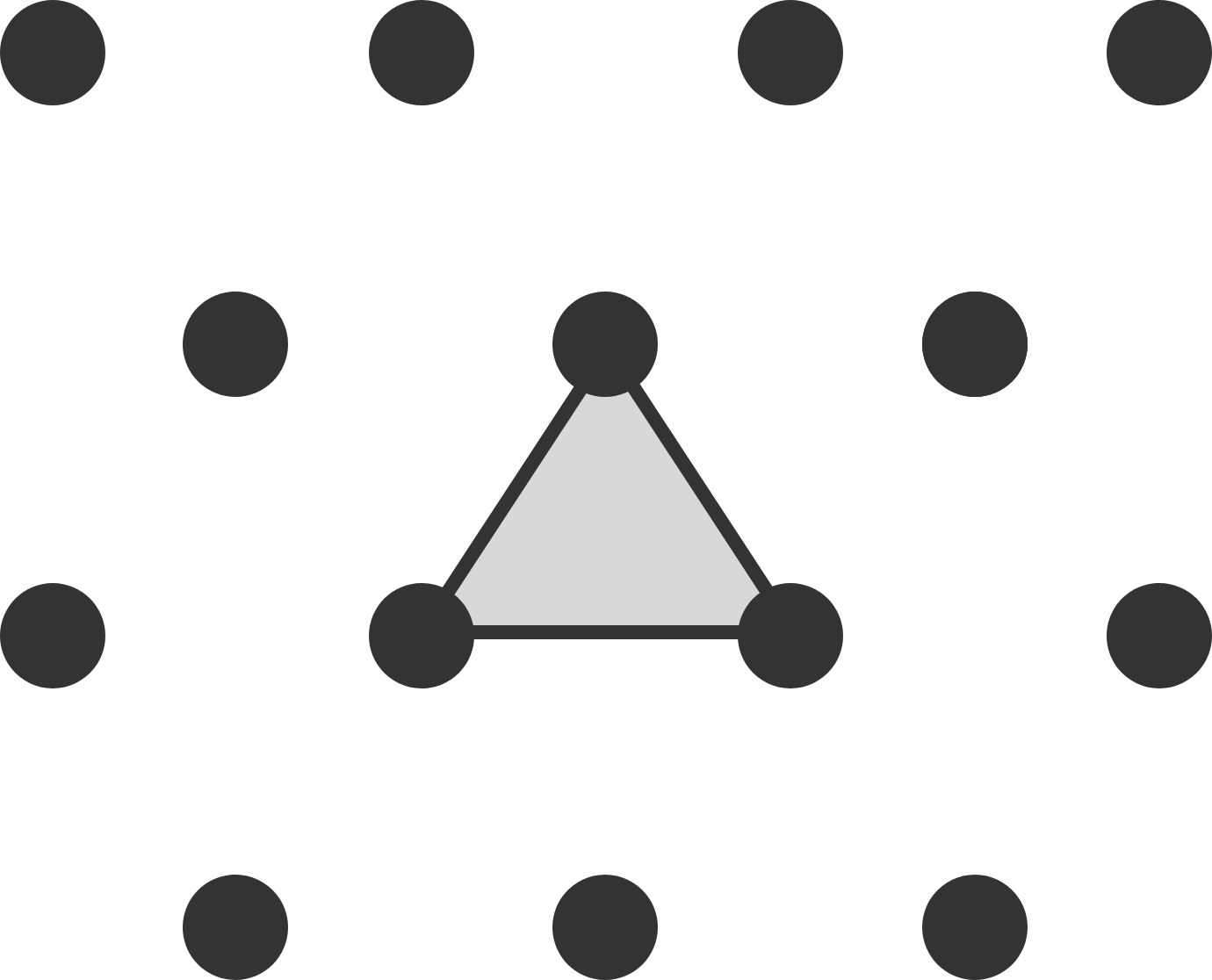# Triangular Pick's Theorem

Geometry Level 2

Pick’s theorem states that, given a polygon with vertices on a unit square lattice, the relationship between the area $A_S$ of the polygon, the number of boundary points $B$ of the polygon, and the number of interior points $I$ of the polygon is $A_S = I + \frac{1}{2}B - 1$.

There exists a similar relationship for a polygon with vertices on an equilateral triangle lattice in which the area of the smallest possible triangle is 1.The area of the shaded triangle is 1.

If $A_T$ is the area of the polygon, $B$ is the number of boundary points of the polygon, and $I$ is the number of interior points of the polygon, then the relationship is $A_T = aI + bB + c.$

What is $|a|+|b|+|c|?$

×